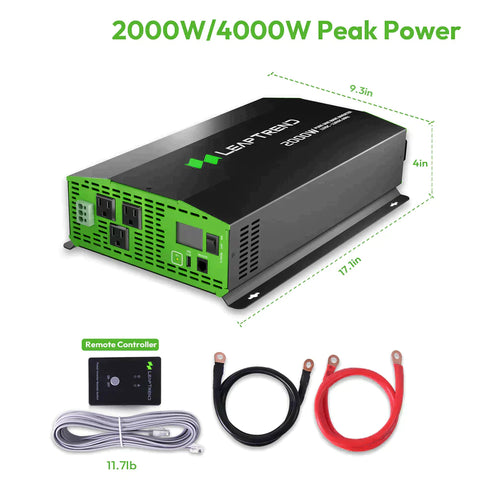# Can a 2000W Inverter Run a Microwave?

In outdoor activities, camping, or during power outages, we often rely on inverters to power our electronic devices. However, for high-power devices like microwaves, it is important to ensure that the inverter's capacity is sufficient to support their operation. In this article, we will answer a common question: Can a 2000W inverter run a microwave? We will provide an explanation and explore the topic in detail.

## Understanding Microwave Power

Firstly, we need to understand the power requirements of the microwave. Different models and sizes of microwaves have varying power consumption, usually measured in watts (W). This information can usually be found in the specifications or labels of the microwave. Let's assume our microwave has a power consumption of 1200 watts.## Inverter Capacity and Efficiency

Next, we need to consider the capacity and efficiency of the inverter. A 2000W power inverter indicates its maximum output power is 2000 watts. However, the actual output power of the inverter may be influenced by its efficiency. Inverter efficiency is typically expressed as a percentage, representing the ratio of input energy converted to output energy. Let's assume our inverter has an efficiency of 90%.

## Calculating if the Inverter Can Run the Microwave

Now, we can calculate if the inverter can run the microwave. Firstly, we need to consider the actual output power of the inverter. Multiply the maximum output power of the inverter by its efficiency, i.e., 2000W * 0.9 = 1800W. This indicates that the actual output power of the inverter is 1800 watts.Next, we compare the actual output power of the inverter with the power requirement of the microwave. If the actual output power of the inverter is greater than or equal to the power requirement of the microwave, then the inverter can run the microwave. In this case, an 1800W inverter can meet the power requirement of a 1200W microwave.

In conclusion, a 2000W inverter can run a microwave with a power consumption of 1200 watts. However, it is important to note that the actual output power of the inverter may be influenced by its efficiency and other factors. Therefore, when selecting an inverter, it is recommended to leave some margin to ensure stable operation of high-power devices.